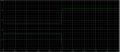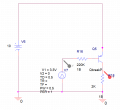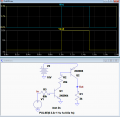# PNP Transistor as a switch

#### sunil0790

Joined Feb 11, 2013
14
Hello All, I am trying to use a PNP transistor as a inverse switch. the output voltage should be 0V & 10V. My base voltage is 0 & 3.3V. The below images are the circuit I have used, but I am not getting O/P as 0V and 10V but something the below waveformThe schematic used is below.Is there anything I could do to increase the O/P voltage to 0V & 10V, with out changing the I/P voltage levels.

#### crutschow

Joined Mar 14, 2008
24,749
Is there anything I could do to increase the O/P voltage to 0V & 10V, with out changing the I/P voltage levels.
Not sure what you want..

If you just want an inverted signal, than use an NPN with the emitter grounded, and the resistor from collector to the 10V supply.

If you want to use a PNP as a high side switch (grounded load), the base voltage has to be less than 0.7V below the supply (emitter) voltage to turn it off.
Typically that's done with an NPN driver transistor.

LTspice simulation of an example circuit is shown below:

Note that when using a BJT as a switch, the base current is typically set to be about 10% of the collector current to insure it is fully on with minimum saturation voltage, which is what the shown resistor values do.
You do not use the Beta transistor current gain values for calculating those resistor values because that gain value is only for calculating base current when the transistor is used in the linear mode, not when it's used as a switch.
(How did you arrive at 220kΩ for the base resistor value?)Last edited:
•atferrari and sunil0790

#### sunil0790

Joined Feb 11, 2013
14
Not sure what you want..

If you just want an inverted signal, than use an NPN with the emitter grounded, and the resistor from collector to the 10V supply.

If you want to use a PNP as a high side switch (grounded load), the base voltage has to be less than 0.7V below the supply (emitter) voltage to turn it off.
Typically that's done with an NPN driver transistor.

LTspice simulation of an example circuit is shown below:

Note that when using a BJT as a switch, the base current is typically set to be about 10% of the collector current to insure it is fully on with minimum saturation voltage, which is what the shown resistor values do.
You do not use the Beta transistor current gain values for calculating those resistor values because that gain value is only for calculating base current when the transistor is used in the linear mode, not when it's used as a switch.
(How did you arrive at 220kΩ for the base resistor value?)

View attachment 199273
Thank you this helps a lot.

#### AnalogKid

Joined Aug 1, 2013
8,384
Q2 base pull-up?

ak

•atferrari and Kjeldgaard